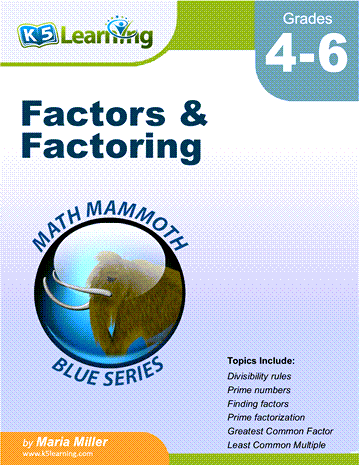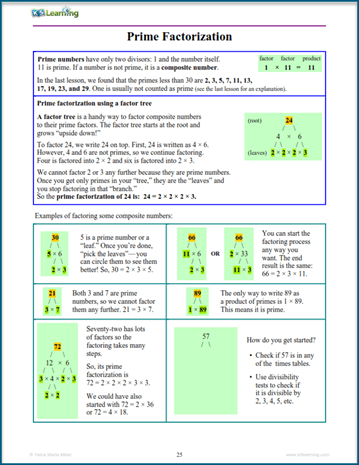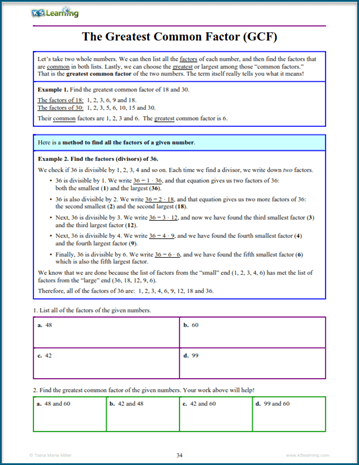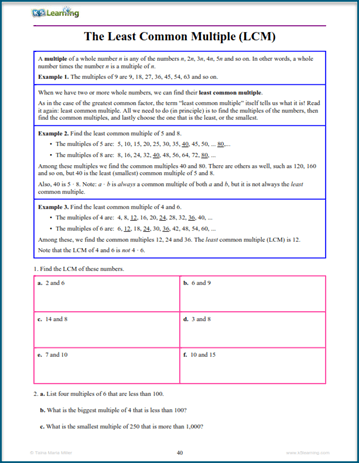# K5 Adds Factors and Factoring Workbook to its Bookstore

We’ve added a new math workbook to our bookstore that covers topics related to factoring and prime numbers.

The workbook is appropriate for kids in grade 4 to 6, depending on when they are taught factors and factoring as part of their curriculum.

## Factors and factoring workbookThis math workbook is divided into 10 sections. Each section starts with a short tutorial with an example, followed by practice exercises. The topics covered in this workbook are:

• divisibility rules;
• prime numbers;
• finding factors;
• factoring sums;
• prime factorization;
• greatest common factors (GCF);
• least common multiples (LCM).

## Sample factoring pages

If you’re curious what the pages look like, look at these sample pages: